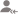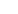truePost a Lesson

All

All

Lessons

Discussion

Lesson Posted on 03/01/2018 SimilarityRaj Kumar

I am Six Sigma Black belt trained from American Society of Quality I am 2011 pass out in B.tech from...

Similar figures and congruent figures both have the same shape. Two triangles are congruent if they are the same size and shape. Congruency:They are exactly same in length, width, shape, size etc. They are similar if they are the same shape (and maybe or maybe not the same size). Also remember: i.... read more

Similar figures and congruent figures both have the same shape.

Two triangles are congruent if they are the same size and shape.

Congruency:They are exactly same in length, width, shape, size etc.

They are similar if they are the same shape (and maybe or maybe not the same size).

Also remember:

i. Every congruent pair of shapes is similar also.

ii. Not all similar figures are congruent though.

iii. The areas of two similar figures may be different.

iv. However, the areas of two congruent figures are equal.

v. Ratio of Corresponding Sides:

a. The ratios between the corresponding sides of two similar figures are equal.

b. The ratios between the corresponding bases of two congruent figures are always one.

vi. Symbol:

a. The symbol ≅ is for Congruent.

b. The symbol ~ is for Similar.

vii. Examples:

You went to a garment store to buy shirts. You liked one with black strips. You saw they were kept in various sizes: S, M, L, XL, XXL…

Now these shirts of different sizes are similar because they have the same shape.

But 2 shirts of the same size are congruent. They are exactly same in length, width, shape, size etc.

Two triangles are said to be similar to each other when they have same shape, proportion and angles but different sizes

Congruence on the other hand means when two things are exactly identical to each other.

The difference between similar figures and congruent figures is that congruent figures also have the same size. So, corresponding sides and corresponding angles of congruent figures have the same measures.Dislike BookmarkSouvik Barua

Btech/BE Tutoring

The SSS rule states that:- If three sides of one triangle are equal to three sides of another triangle, then the triangles are congruent. In the diagrams below, if AB = RP, BC = PQ and CA = QR, then triangle ABC is congruent to triangle RPQ. Side - Side - Side (SSS) Rule Side-Angle-Side (SAS) Rule Side-Angle-Side... read more
The SSS rule states that:- If three sides of one triangle are equal to three sides of another triangle, then the triangles are congruent. In the diagrams below, if AB = RP, BC = PQ and CA = QR, then triangle ABC is congruent to triangle RPQ. Side - Side - Side (SSS) Rule Side-Angle-Side (SAS) Rule Side-Angle-Side is a rule used to prove whether a given set of triangles are congruent. The SAS rule states that :- If two sides and the included angle of one triangle are equal to two sides and included angle of another triangle, then the triangles are congruent. An included angle is an angle formed by two given sides. Included and Non-included angle:- Included Angle Non-included angle For the two triangles below, if AC = PQ, BC = PR and angle C = angle P , then using the SAS rule, triangle ABC is congruent to triangle QRP congruent triangles Angle-Side-Angle (ASA) Rule Angle-side-angle is a rule used to prove whether a given set of triangles are congruent. The ASA rule states that If two angles and the included side of one triangle are equal to two angles and included side of another triangle, then the triangles are congruent. Angle-Angle-Side (AAS) Rule Angle-angle-side is a rule used to prove whether a given set of triangles are congruent. The AAS rule states that If two angles and a non-included side of one triangle are equal to two angles and a non-included side of another triangle, then the triangles are congruent. In the diagrams below, if AC = QP, angle A = angle Q, and angle B = angle R, then triangle ABC is congruent to triangle QRP. read less
Dislike BookmarkSujoy D.

Tutor

There are the following steps to prove that two triangles are similar:- 1. AAA Test (angle angle angle): All three pairs of corresponding angles are the same. 2. SSS Test (side side side): All three pairs of corresponding sides are in the same proportion. 3. SAS Test (side angle side): Two pairs of sides... read more

There are the following steps to prove that two triangles are similar:- 1. AAA Test (angle angle angle): All three pairs of corresponding angles are the same. 2. SSS Test (side side side): All three pairs of corresponding sides are in the same proportion. 3. SAS Test (side angle side): Two pairs of sides are in the same proportion and the angle between them is equal.

Dislike BookmarkShanta Kothari

Complementary angles are the angles which add up to 90 and supplementary angles are the angles that add up to 180 .
Dislike BookmarkSujoy D.

Tutor

Two triangles are similar if they have: -All their angles equal. -Corresponding sides are in the same ratio.
Dislike BookmarkSujoy D.

Tutor

3 main criteria for similarity of triangles 1) AAA or AA 2) SSS 3) SAS.
Dislike BookmarkSujoy D.

Tutor

In a right angled triangle the square of the long side is equal to the sum of the squares of the other two sides. It is stated in this formula: a2 + b2 = c2
Dislike BookmarkAnkit

JEE/Board Maths Tutor

If one angle between two adjacent sides of a triangle is similar to angle between two sides of another triangle then two triangles are congruent.
Dislike BookmarkManish V

Tutor

SSS,SAS,AA
Dislike BookmarkJayam Sagar

Home Tutor

Yes
Dislike Bookmark

UrbanPro.com helps you to connect with the best in India. Post Your Requirement today and get connected.16 Followers
x

X

### Find Tutors, Trainers & Institutes near you

Post requirement and connect with the tutors in your locality

• Post a learning requirement
• Get customized responses
• Compare and select the best### Want to learn something New?

Find best tutors, trainers & institutes near you on UrbanProUrbanPro.com is India's largest network of most trusted tutors and institutes. Over 25 lakh students rely on UrbanPro.com, to fulfill their learning requirements across 1,000+ categories. Using UrbanPro.com, parents, and students can compare multiple Tutors and Institutes and choose the one that best suits their requirements. More than 6.5 lakh verified Tutors and Institutes are helping millions of students every day and growing their tutoring business on UrbanPro.com. Whether you are looking for a tutor to learn mathematics, a German language trainer to brush up your German language skills or an institute to upgrade your IT skills, we have got the best selection of Tutors and Training Institutes for you. Read more